### Equilateral Triangle Inscribed In An Equilateral Triangle

Illustration used to prove that triangle EFD is equilateral given that triangle ABC is equilateral and…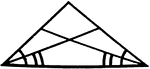### Angle Bisectors In An Isosceles Triangle

Illustration showing that if two angles of a triangle are equal, the bisectors of these angles are equal.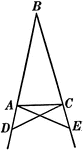### Base Angles In An Isosceles Triangle

Illustration showing that can be used to prove that the base angles of an isosceles triangle are equal.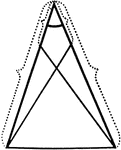### Equal Segments In An Isosceles Triangle

Illustration showing that if equal segments measured from the vertex are laid off on the arms of an…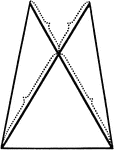### Equal Segments In An Isosceles Triangle

Illustration showing that if equal segments prolonged through the vertex are laid off on the arms of…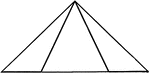### Equal Segments In An Isosceles Triangle

Illustration showing that if equal segments measured from the end of the base are laid off on the base…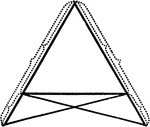### Equal Segments In An Isosceles Triangle

Illustration showing that if equal segments measured from the end of the base prolonged are laid off…